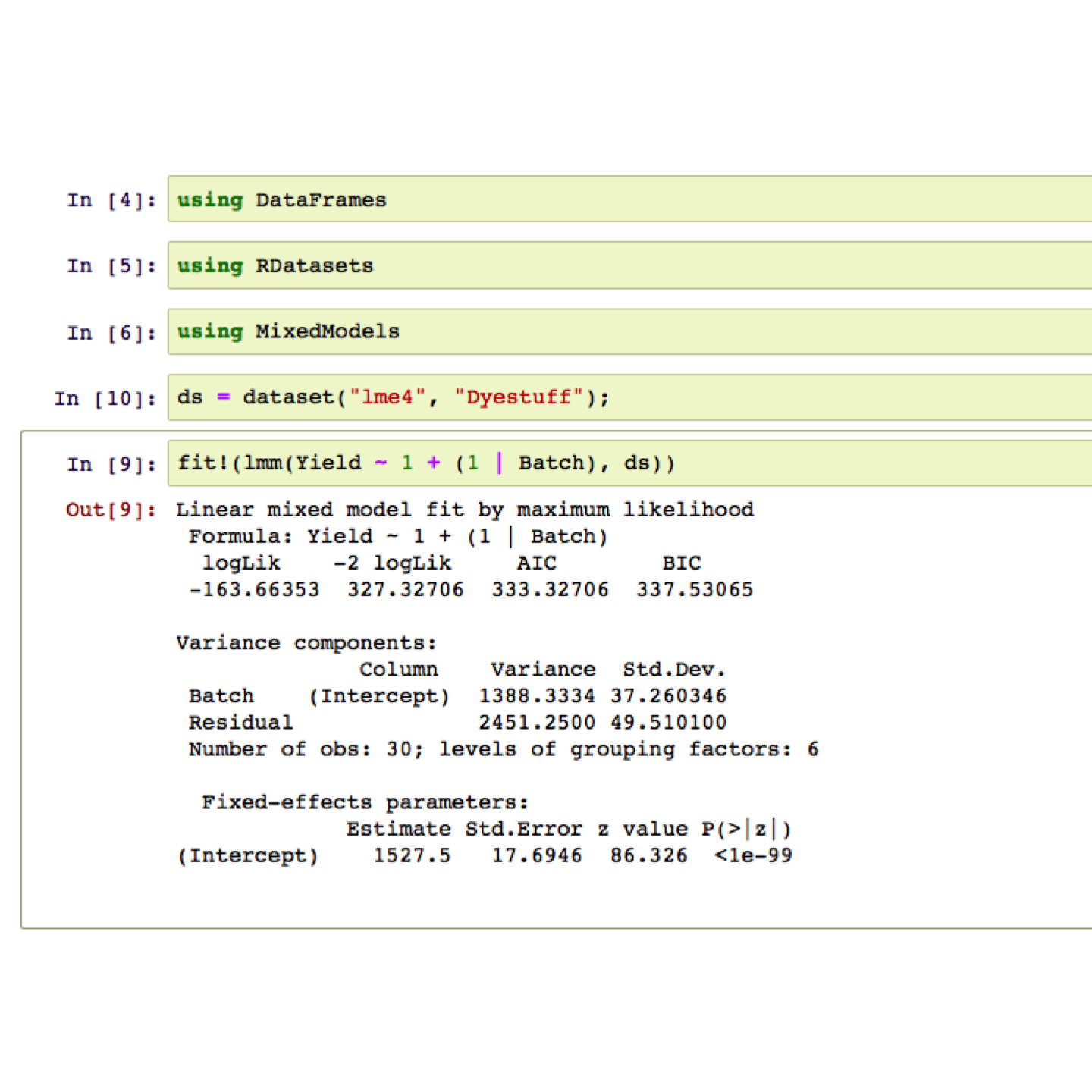# Julia for R users (written in Rstudio)

Hannah Lennon
16th November 2016

### Julia is

• a high-level, high-performance dynamic programming language

• for technical computing, with syntax that is familiar to other computing environments

• The library, mostly written in Julia itself, also integrates mature, best-of-breed C and Fortran libraries for linear algebra, random number generation, FFTs, and string processing.

• It provides a sophisticated compiler, distributed parallel execution, numerical accuracy, and an extensive mathematical function library.

### What I think Julia is

• Free

• The future

• FAST (~2–3x C speed)

• Well thoughtout (is JIT compiled & everthing in Julia is an expression)

• Uses syntax almost identical to Matlab (very similar to R)

• Written by mathematicians, statisticians and computer scientists for mathematicians, statisticians and computer scientists

• Overall, deals with the two-language problem

### My favourite bits

• The architecture has been so well though through

1. Write code as you code up your research e.g. mu <- sum(x)/length(x)
1. Delve down a level to see how the computer is implementing, then delve some more, and more and even to the binary 0, 1's of your hardware
1. To allow high level programming with all of the deep, dark aspects some of which are seen using C++, Fortran
• You are in control when you want to be, and when you don't you can rest assured Julia will be quicker than R

• Oh and you can call R from Julia

### Julia from the command line

• Using the ; key switches from Julia in the command line to my bash shell

• Structure similar to python (Pkg.add(“X”) and using X)

### Example 1: Linear mixed effect model in R

library(lme4)

  Batch Yield
1     A  1545
2     A  1440
3     A  1440
4     A  1520
5     A  1580
6     B  1540

lmer(Yield ~ 1|Batch, Dyestuff, REML=FALSE)

Linear mixed model fit by maximum likelihood  ['lmerMod']
Formula: Yield ~ 1 | Batch
Data: Dyestuff
AIC       BIC    logLik  deviance  df.resid
333.3271  337.5307 -163.6635  327.3271        27
Random effects:
Groups   Name        Std.Dev.
Batch    (Intercept) 37.26
Residual             49.51
Number of obs: 30, groups:  Batch, 6
Fixed Effects:
(Intercept)
1527


### Example 1: Linear mixed effect model in Julia### Survival Example 2: Cox regression in R

library(survival)
data("ovarian")

  futime fustat     age resid.ds rx ecog.ps
1     59      1 72.3315        2  1       1
2    115      1 74.4932        2  1       1
3    156      1 66.4658        2  1       2
4    421      0 53.3644        2  2       1
5    431      1 50.3397        2  1       1
6    448      0 56.4301        1  1       2

ovarian$group <- ovarian$rx - 1
coxph(Surv(futime, fustat) ~ age + group, data=ovarian)

Call:
coxph(formula = Surv(futime, fustat) ~ age + group, data = ovarian)

coef exp(coef) se(coef)     z      p
age    0.1473    1.1587   0.0461  3.19 0.0014
group -0.8040    0.4475   0.6320 -1.27 0.2034

Likelihood ratio test=15.9  on 2 df, p=0.000355
n= 26, number of events= 12